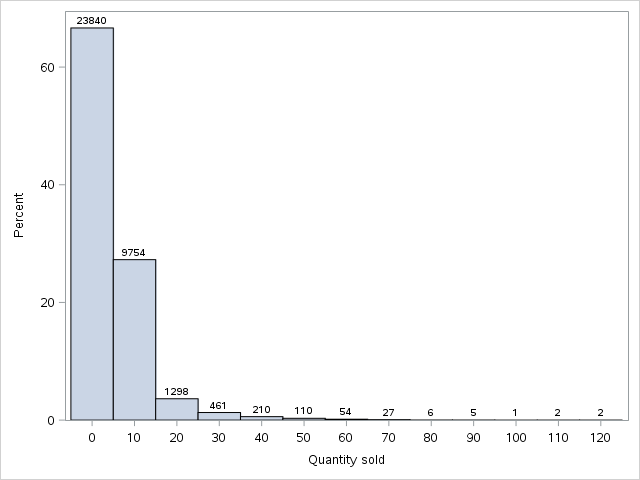## SGPLOT error on Histogram counts

Using SAS 9.04.01 M3 on Windows 7, with EG 7.1 on a Linux Grid.

Counts are off by 7046 on first bin of histogram as compared to proc means. Values are 0 to 10. What am I missing? 30886-23840 = 7046

 ``````title "Snacks test - All Qtysold bins 0 to 10"; proc sgplot data=sashelp.snacks; histogram qtysold/datalabel=count scale=percent showbins binstart=0 binwidth=10 boundary=lower; run; title "qtysold <11"; proc means data=sashelp.snacks(where=(0 le qtysold le 10)) n nmiss min p25 median p75 max mean ; var qtysold; run;``````SAS Output
 qtysold <11

The MEANS Procedure

Analysis Variable : QtySold Quantity sold
N N Miss Minimum 25th Pctl Median 75th Pctl Maximum Mean
 30886 0 0 0 2 5 10 2.96445
1 ACCEPTED SOLUTION

Accepted Solutions

## Re: SGPLOT error on Histogram counts

``````title "Snacks test Scale=pct Qtysold bins 0 to 10";
proc sgplot data=sashelp.snacks(where=(0 le qtysold le 100));
histogram qtysold/datalabel=count showbins scale=percent binstart=5 binwidth=10 boundary=lower;
run;
title "qtysold <=10";
proc means data=sashelp.snacks(where=(0 le qtysold le 10)) n nmiss min p25 median p75 max mean ;
var qtysold;
run;
title "qtysold 11? to 20 - non-integers in qtysold variable";
proc means data=sashelp.snacks(where=(10 lt qtysold le 20)) n nmiss min p25 median p75 max mean ;
var qtysold;
run;

``````

This works. I'm still not sure how to control the first bin.

4 REPLIES 4

## Re: SGPLOT error on Histogram counts

The boundaries used for this histogram with your options are 5, 15, 25, 35. Binstart does not actually set a boundary, it more associated with the displayed x value which would be the center of the bar range shown. Hence 10 is mid-point of the 5-15 range.

If you really have to force displays to intervals of 0-10, 10-20 then use VBAR and a format to create ranges for the xaxis variable.

## Re: SGPLOT error on Histogram counts

Why are zero values of qtysold ignored on histogram? Where is the first bin controlled so it is 5 to 15? What about 0,1,2,3,4 values?

## Re: SGPLOT error on Histogram counts

``````title "Snacks test Scale=pct Qtysold bins 0 to 10";
proc sgplot data=sashelp.snacks(where=(0 le qtysold le 100));
histogram qtysold/datalabel=count showbins scale=percent binstart=5 binwidth=10 boundary=lower;
run;
title "qtysold <=10";
proc means data=sashelp.snacks(where=(0 le qtysold le 10)) n nmiss min p25 median p75 max mean ;
var qtysold;
run;
title "qtysold 11? to 20 - non-integers in qtysold variable";
proc means data=sashelp.snacks(where=(10 lt qtysold le 20)) n nmiss min p25 median p75 max mean ;
var qtysold;
run;

``````

This works. I'm still not sure how to control the first bin.

## Re: SGPLOT error on Histogram counts

If you actually want a vertical bar chart with the width at points you control then use VBAR and a format to create groups

```proc format library=work;
value bins
0  - 10 = ' 0'
10 <- 20 = '10'
20 <- 30 = '20'
30 <- 40 = '30'
40 <- 50 = '40'
50 <- 60 = '50'
60 <- 70 = '60'
70 <- 80 = '70'
80 <- 90 = '80'
90 <-100 = '90'
;
run;
proc sgplot data=sashelp.snacks(where=(0 le qtysold le 100));
vbar qtysold/stat=freq datalabel;
format qtysold bins.;
run;
```
Discussion stats
• 4 replies
• 1037 views
• 0 likes
• 2 in conversation